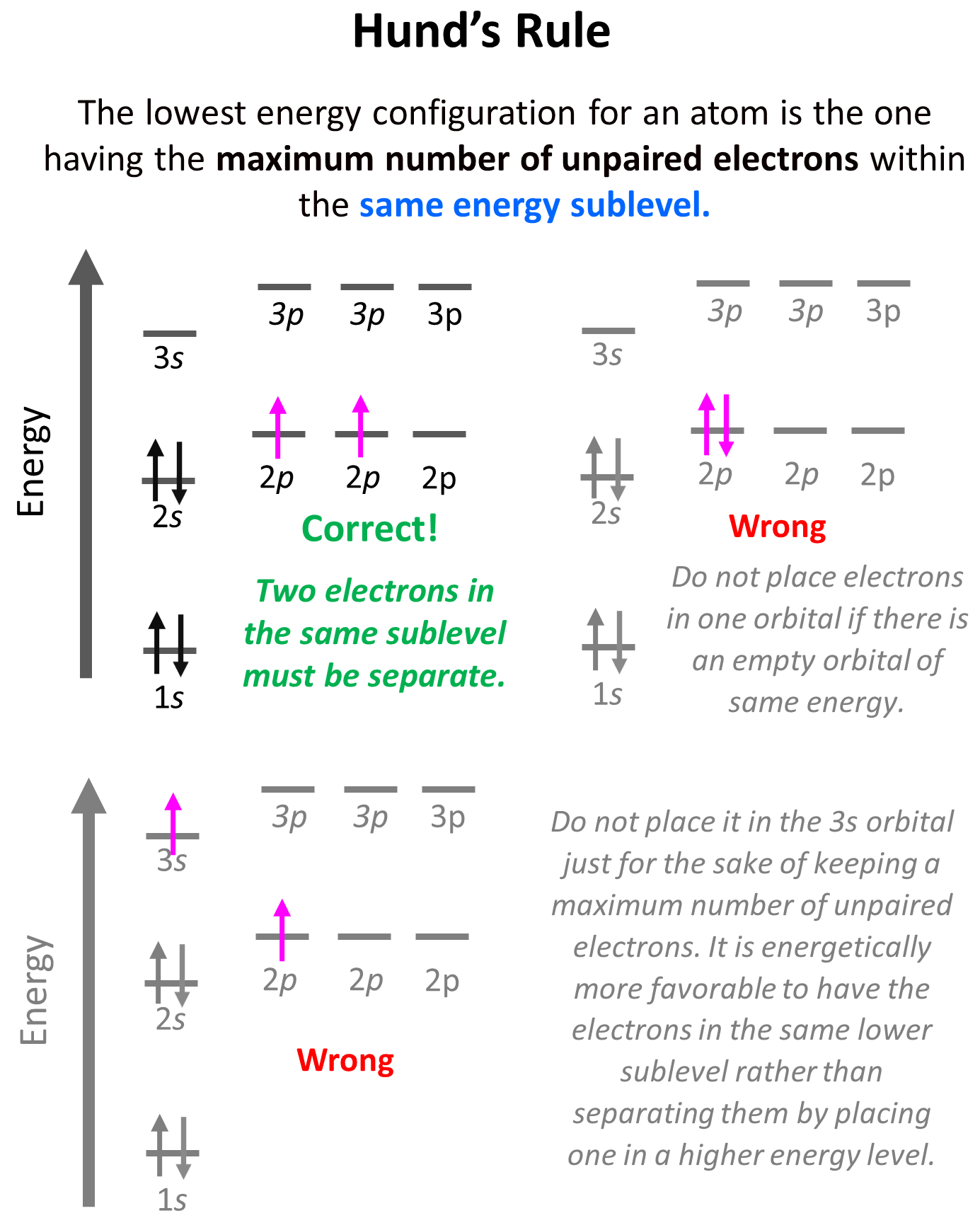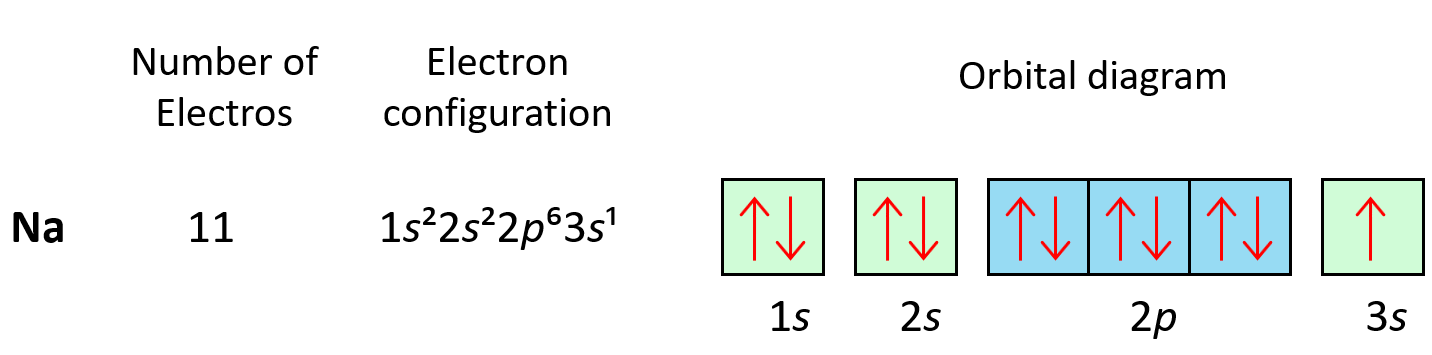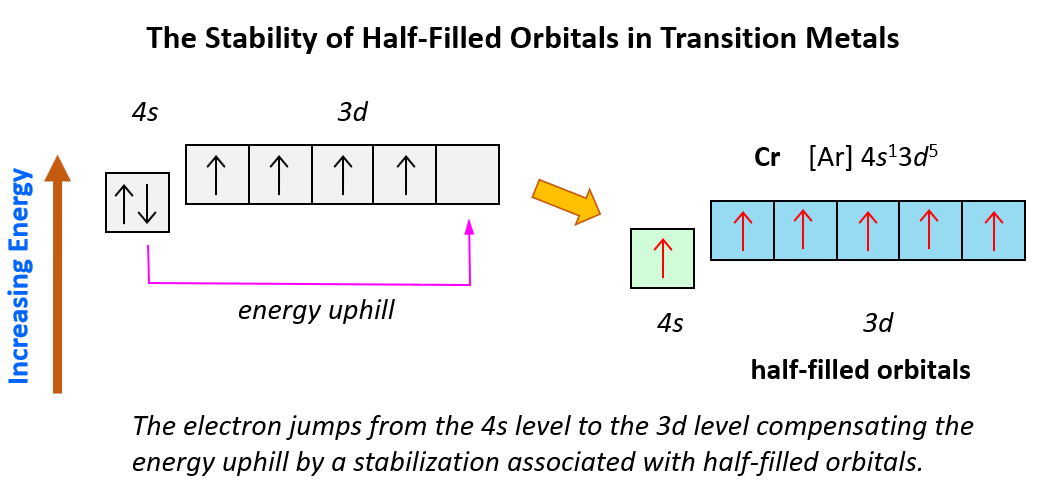## General Chemistry

Hund’s rule states that electrons will fill all the degenerate orbitals (equal in energy) with parallel spins (both arrows up or down) first before pairing up in one orbital. We can also formulate it as the lowest energy configuration for an atom is the one having the maximum number of unpaired electrons within the same energy sublevel.

To understand Hund’s tule, let’s first write the electron configuration of boron using an orbital diagram:Now, the next election, which indicates carbon, is going to have the option of pairing up with the one in the p orbital or going to the next empty p orbital. And it turns out that the electron goes to the next (emptyp orbital rather than fitting in with the other electron.Hund’s rule is another demonstration of the same principle which is the tendency to adopt the lowest energy state possible. There is a stronger repulsive interaction between two electrons in the same orbital compared to when they occupy separate orbitals of equal energy. However, keep in mind that having unpaired electrons is the most important factor in keeping the electrons in lowest energy level. First, we have the main energy levels, given by the principal quantum number (n), and the orbitals are filled according to the Aufbau principle. For example, going back to the electron configuration of carbon, notice that we did not place the second electron in the 3s orbital:Placing the electron unpaired in the 3s orbital is incorrect because, it is important to mention, that Hund’s rule applies to electrons in the same energy level. We are not going to place one of the electrons in the 3s orbital just for the sake of keeping a maximum number of unpaired electrons. It is energetically more favorable to have the electrons in the same lower sublevel rather than separating them by placing one in a higher energy level.

As expected, the next element, nitrogen will place the additional electron in the 3rd p orbital which is empty in carbon:The next three elements fill the p orbitals according to the Pauli’s exclusion principle before sodium puts the next electron in the 3s level.Some exceptions, where the electron is placed unpaired in a higher energy orbital, occur in the electron configuration of d and f elements. First, recall that the (n +1)s orbitals always fill before the nd orbitals. For example, the 4s level fills before the 3d level, and therefore, the electron configuration of Ti is 1s²2s²2p3s²3p4s²3d² or, the condensed configuration which will be [Ar]4s²3d².And now, let’s look at the configuration of chromium: Unlike the expected [Ar]4s²3d4, the electron configuration of Cr is [Ar]4s13d5, and the reason for this is that the d orbital gets a half-filled configuration (remember d orbitals can have a maximum of 10 electrons). We can think of this as the electron jumping from the 4s level to the 3d level and compensating this energy uphill by a stabilization associated with half-filled orbitals:Again, this is due to lowering energy when the atom attains half-filled orbitals which goes along the Hund’s rule.

Check Also